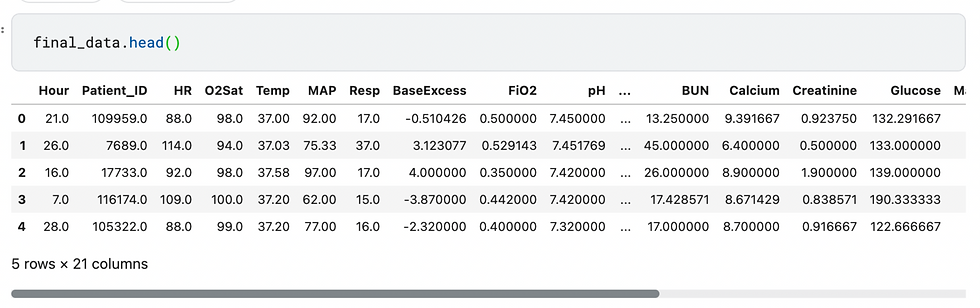top of pageSearch

# Under Sampling

Handling data is one of the major tasks in data science. And while working on datasets it’s quite common for us to work on imbalanced data. To balance data we have two methods called under sampling and over sampling. They are also called as resampling. Let us imagine we have a dataset which we are planning to test and train. But to find the accuracy if we use the imbalanced data the result may not be perfect. Oversampling and Undersampling in data analysis are techniques used to adjust the class distribution of a data set. These terms are used both in statistical sampling, survey design methodology and in machine learning. Resampling methods are designed to add or remove examples from the training dataset in order to change the class distribution. Once the class distributions are more balanced, the suite of standard machine learning classification algorithms can be fit successfully on the transformed datasets.

Under sampling :

Under sampling can be defined as a technique to balance uneven datasets by keeping all of the data in the minority class and decreasing the size of the majority class. It is one of the several techniques data scientists use to extract more accurate information from originally imbalanced datasets.

Under sampling removes samples from the majority class, with or without replacement. Oversampling involves supplementing the training data with multiple copies of some of the minority classes. To overcome this issue under sampling or over sampling is used. Let us imagine we are training dataset with X which have 100 rows and y with 10 rows. If we do under sampling then the based on the code, it picks randomly 10 rows from X and the accuracy is given based on the 10 rows from X and 10 rows from y total for 20 rows.

Over sampling :

Oversampling methods duplicate or create new synthetic examples in the minority class. For Machine Learning algorithms affected by skewed distribution, such as artificial neural networks and SVMs, this is a highly effective technique.

In Oversampling let us imagine we are training dataset with X which have 100 rows and y with 10 rows, the 10 rows in y will create its duplicate rows and will become 100 rows, then the oversampling is performed on the 100 rows of X and 100 rows of y. If there is a huge difference between X and y rows , it’s always better to perform Oversampling, so that the accuracy will be given for all 200 rows. In extreme cases where the number of observations in the rare class(es) is really small, oversampling is better, as you will not lose important information on the distribution of the other classes in the dataset.

Let‘s go through the example of under sampling :

1. Importing Libraries :

```import numpy as np
import pandas as pd
from sklearn.impute import KNNImputer
from sklearn.model_selection import train_test_split, StratifiedKFold
from sklearn.ensemble import RandomForestClassifier
from xgboost import XGBClassifier
from sklearn.datasets import make_classification\
from sklearn.impute import SimpleImputer
import plotly.graph_objs as go
import plotly.offline as py
import seaborn as sns
import matplotlib.pyplot as plt
from sklearn.metrics import f1_score
from sklearn.metrics import mean_squared_error
from sklearn import metrics
from sklearn.metrics import roc_auc_score
from sklearn.metrics import accuracy_score
from sklearn.metrics import mean_absolute_error
from sklearn.metrics import precision_score
from imblearn.under_sampling import RandomUnderSampler```

`df1=pd.read_csv("../input/sepsis50/sepsis_50p.csv")`

3. Making sure there are no null values for required columns

`df1.isnull().sum()`4. Checking the count of data based on the target column

`print(sorted(Counter(df1['SepsisLabel']).items()))`5. Inserting the datasets into X, y

```X=df1
y=df1['SepsisLabel']```

`X.drop('SepsisLabel',axis=1, inplace = True)`

6. Installing imblearn and importing under sampling

```# pip install imblearn

from imblearn import under_sampling, over_sampling```

After importing RandomUnderSampler, fitting the X value and y value into X_sampled, y_resampled.

```from imblearn.under_sampling import RandomUnderSampler
rus = RandomUnderSampler(random_state=0)
X_resampled, y_resampled = rus.fit_resample(X,y)
print(sorted(Counter(y_resampled).items()),y_resampled.shape)```7. Considering the X_sampled as an df

```from numpy import mean
from sklearn.datasets import make_classification
from sklearn.model_selection import cross_val_score
from sklearn.model_selection import RepeatedStratifiedKFold
from sklearn.tree import DecisionTreeClassifier
from imblearn.pipeline import Pipeline```

```df2 = pd.DataFrame(X_resampled)
df2```8. Fitting KNNImputer to X_sampled(df2) dataset

```print
imputer = KNNImputer()
imp_data = imputer.fit_transform(df2)
column_names = list(df2)
final_data = pd.DataFrame(imp_data, columns = column_names)```

`final_data.head()`9. Considering the imputed dataset as X1, train and test X1 and y datasets

```X1 = final_data
y= y_resampled```

`y_resampled.head()`

`X1_train,X1_test,y1_train,y1_test = train_test_split(X1,y,test_size = 0.30,random_state = 100,stratify = y)`10. Here we are using RandomForestClassifier to predict the results. A random forest is a meta estimator that fits a number of decision tree classifiers on various sub-samples of the dataset and uses averaging to improve the predictive accuracy and control over-fitting. We can test with other models for better results.

```
classifier = RandomForestClassifier(n_estimators = 40, random_state=100)
rfc=classifier.fit(X1_train, y1_train)
# Predicting the test set results
y_pred = classifier.predict(X1_test)```

11. Checking the f1 score using RandomForestClassifier model. F1 score can be considered as perfect when it is 1. And model is a total failure when it’s 0.

`rfc.score(X_test,y_test)`

```from sklearn.metrics import classification_report
print(classification_report(y_test, y_pred))```From the above predictions we got 0.92 f1 score. It is a good score and we can proceed with under sampled data.

we can conclude that Random oversampling involves randomly duplicating examples in the minority class, whereas random undersampling involves randomly deleting examples from the majority class.